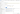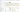## Create Aha! scorecard equations

The Aha! scorecard can be used to prioritize features, requirements, initiatives, and ideas and to rank competitors. It can also be added as a custom field across Aha! and as a field identifier in custom equation functions.

You can create multiple scorecards intended for multiple workspaces or record types.

A scorecard is made up of metrics. For features and ideas, each metric should reflect some aspect of your strategy to ensure you can use it to objectively measure feature or idea value. This document details how you can set up a scorecard.

The Aha! score equation editor allows users to define an equation to calculate the scores of features and ideas that use the scoring system. Scores are calculated using some combination of user-defined metrics.

To add, edit, clone or delete scorecards, navigate to Settings ⚙️→ Account → Configure scorecards. Click on a scorecard name or click the Add scorecard button to open the scorecard configuration screen.Add new metrics by clicking the blue Add Metric button. Click the Edit button by a metric to adjust it.

• Sequence Type: This dropdown allows you to choose the type of numerical sequence you prefer for this metric.

• Linear: Values increment linearly (1,2,3,4,5)

• Powers of 2: Values increment by a power of 2 (0,1,2,4,8,16)

• Fibonacci Sequence: Values increment based on the sum of the previous two (0, 1, 2, 3, 5, 8, 13)

• Custom: Define your own custom sequence type

• Value Range: This defines the range of values available to select when scoring the metric.

• Step Value: This field allows the linear sequence type to have a customized step value, such as incrementing by 5 (e.g. 5, 10, 15, etc.).

• Units: This field allows you to append or prepend a unit type to your scorecard units, such as \$, %, or hours.

Top

## Create an equation

As you edit the bounds of a metric, an example slider will be generated in the scorecard's Preview box. You may test your equation by manipulating these example sliders; the score of a hypothetical record with the assigned metric values is displayed at the bottom of the preview. Note that scores with decimal values will be rounded up to the nearest whole number.

The editor provides two modes to create your equation: Simple and Advanced. In simple mode, the equation is defined as the sum of metric values, each optionally multiplied by a constant. Click on each constant in the equation to type a new numerical value.Top

In advanced mode, you may define your own custom equation using numbers, mathematical operators, and metric values. Supported mathematical operations are:

• Subtraction (-)

• Multiplication (*)

• Division (/)

• Modulo (%)

• Exponentiation (^)

• Parentheses ()

Note: Outside of brackets, the equation will follow a standard PEMDAS order of operations.

You may also use the following functions in your custom equation.

• If - if(expression, true_value, false_value) - evaluates the expression. It uses true_value if the expression is true or false_value if it is false. For example, if(3 >2, 10, 20) would evaluate to 10. You may use metrics in any parameter- for example, if(Difficulty >Added Value, -1 * Difficulty, Added Value). Valid expression operators are >, >=, <, <=, and =.

• Round - round(number, decimals) - rounds the number to the provided number of decimal places. If no decimals parameter is provided, the function rounds to the nearest whole number. For example, round(8.2759, 2) evaluates to 8.28, while round(8.75) evaluates to 9.*

• Roundup - roundup(number, decimals) - is identical to round, except it always rounds up. For example, roundup(8.25) evaluates to 9.*

• Rounddown - rounddown(number, decimals) - is identical to round, except it always rounds down. For example, rounddown(8.75) evaluates to 8.*

Note: The rounding functions may be used to round components of the equation, but the final resulting score will always be a whole number.

To add a metric value, begin typing the metric's name and select it from the autocomplete list. The metric will then be added to the equation, denoted by a box with a yellow background.

The advanced equation editor gives constant feedback so you can ensure that your equation has valid syntax. When the current equation is valid, the editor is outlined in green; when the current equation has an error, the editor is outlined in red. The most common source of equation errors is stray text in the equation; there should never be any letters in an equation outside of metric boxes or functions. The equation will automatically be saved as you type, but only when the equation has valid syntax.

Top

## Map custom scorecards in Aha! integrations

If you send records from Aha! to other tools, it makes sense to send your new custom scorecard values along with those records. In the Mapping step of an Aha! integration, click Field mapping to map your custom scorecard field to an analogous field in the integrated tool.

Top